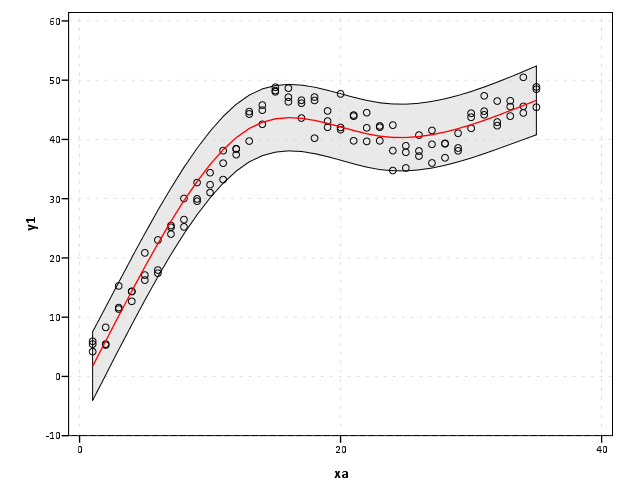# SPSS Statistics

View Only

## Restricted cubic splines in SPSS

####By Archive User posted Thu June 06, 2013 09:41 AM

I've made a macro to estimate restricted cubic spline (RCS) basis in SPSS. Splines are useful tools to model non-linear relationships. Splines are useful exploratory tools to model non-linear relationships by transforming the independent variables in multiple regression equations. See Durrleman and Simon (1989) for a simple intro. I've largely based my implementation around the various advice Frank Harell has floating around the internet (see the `rcspline` function in his HMisc R package), although I haven't read his book (yet!!).

So here is the SPSS MACRO, and below is an example of its implementation. It takes either an arbitrary number of knots, and places them at the default locations according to quantiles of x's. Or you can specify the exact locations of the knots. RCS need at least three knots, because they are restricted to be linear in the tails, and so will return k - 2 bases (where k is the number of knots). Below is an example of utilizing the default knot locations, and a subsequent plot of the 95% prediction intervals and predicted values superimposed on a scatterplot.

``FILE HANDLE macroLoc /name = "D:TempRestricted_Cubic_Splines".INSERT FILE = "macroLocMACRO_RCS.sps".*Example of there use - data example taken from http://www-01.ibm.com/support/docview.wss?uid=swg21476694.dataset close ALL.output close ALL.SET SEED = 2000000.INPUT PROGRAM.LOOP xa = 1 TO 35.LOOP rep = 1 TO 3.LEAVE xa.END case.END LOOP.END LOOP.END file.END INPUT PROGRAM.EXECUTE.* EXAMPLE 1.COMPUTE y1=3 + 3*xa + normal(2).IF (xa gt 15) y1=y1 - 4*(xa-15).IF (xa gt 25) y1=y1 + 2*(xa-25).GRAPH/SCATTERPLOT(BIVAR)=xa WITH y1.*Make spline basis.*set mprint on.!rcs x = xa n = 4.*Estimate regression equation.REGRESSION  /MISSING LISTWISE  /STATISTICS COEFF OUTS R ANOVA  /CRITERIA=PIN(.05) POUT(.10) CIN(95)  /NOORIGIN  /DEPENDENT y1  /METHOD=ENTER xa  /METHOD=ENTER splinex1 splinex2  /SAVE PRED ICIN .formats y1 xa PRE_1 LICI_1 UICI_1 (F2.0).*Now I can plot the observed, predicted, and the intervals.GGRAPH  /GRAPHDATASET NAME="graphdataset" VARIABLES=xa y1 PRE_1 LICI_1 UICI_1  /GRAPHSPEC SOURCE=INLINE.BEGIN GPL SOURCE: s=userSource(id("graphdataset")) DATA: xa=col(source(s), name("xa")) DATA: y1=col(source(s), name("y1")) DATA: PRE_1=col(source(s), name("PRE_1")) DATA: LICI_1=col(source(s), name("LICI_1")) DATA: UICI_1=col(source(s), name("UICI_1")) GUIDE: axis(dim(1), label("xa")) GUIDE: axis(dim(2), label("y1")) ELEMENT: area.difference(position(region.spread.range(xa*(LICI_1+UICI_1))), color.interior(color.lightgrey), transparency.interior(transparency."0.5")) ELEMENT: point(position(xa*y1)) ELEMENT: line(position(xa*PRE_1), color(color.red))END GPL.``See the macro for an example of specifying the knot locations. I also placed functionality to estimate the basis by groups (for the default quantiles). My motivation was partly to replicate the nice functionality of ggplot2 to make smoothed regression estimates by groups. I don't know off-hand though if having different knot locations between groups is a good idea, so caveat emptor and all that jazz.

I presume this is still needed functionality in SPSS, but if this was not needed let me know in the comments. Other examples are floating around (see this technote and this Levesque example), but this is the first I've seen of implementing the restricted cubic splines.

#datavisualization
#MACRO
#SPSS
#SPSSStatistics
#Visualization
0 views

Thu September 21, 2017 01:22 PM

Whoops, gave the wrong link, here is the link to the restricted cubic spline code. https://dl.dropboxusercontent.com/s/xndrv8vgx12v6gd/MACRO_RCS.sps?dl=0

Thu September 21, 2017 01:06 PM

Yes, Dropbox changed my links. An updated link to that macro is here, https://dl.dropboxusercontent.com/s/l5jt8me450fdges/RocClass.sps?dl=0.

If you go to my personal website I have many of the links updated, https://andrewpwheeler.wordpress.com/code-snippets-toc/, but I am probably missing a few.

Sun September 17, 2017 12:52 PM

Is the macro still available? When I click on the hyperlink, nothing happens. Thanks.

Thu July 03, 2014 02:31 PM

This message was posted by a user wishing to remain anonymous
[…] accomplish the same thing in SPSS you can estimate restricted cubic splines and then use any applicable regression procedure (e.g. LOGISTIC, GENLIN) and save the predicted […]

Sat May 10, 2014 07:53 AM

Yes it can be applied to any type of regression model. All the code does is make a set of basis functions, so it turns the original variable into several new variables. These new variables (plus the original variable) should be included on the right hand side for whatever regression equation you are using.

Sat May 10, 2014 07:47 AM

Dear Andrew,

Thanks for sharing this syntax. Do you know if it could be modified to work with Cox regression?

Best wishes.
-Clinton

Wed September 11, 2013 03:24 PM

Hi Thomas,

The macro doesn't have anything to do directly with making graphs. The macro just returns a set of separate variables that are the spline basis, and can be used as independent variables in any regression (be it linear or logistic or whatever).

To recreate a similar chart to what I show for OLS, you could use the GENLIN command to save the lower and upper confidence intervals (to recreate prediction intervals it looks like you will have to calculate your own - but the save commands have all the necessary ingredients). The scatter points just end up being less revealing because all of the points are at two locations on the Y axis (either 0 or 1). People often use jittering and/or rug plots in those circumstances.

If you have any other questions feel free. I figure a post on the type of chart viz. logistic regression would be a good topic and is somewhere in the to-do list, although who knows when I will have time to get to it.

Wed September 11, 2013 02:54 PM

Dear Andrew,

Thanks for the macro.

I'm curretly evaluating the usefulness of splines on one of my PhD projects and using a STATA syntax for that. Since I'm much more used to SPSS this really comes in handy.

I do have a question though. Since the graph takes advantage of the "ICIN" option for the regression, I cannot easily (to my knowledge) reproduce the same kind of graph for a logistic regression. With this, my question: is there a way to adapt the macro to run with logistic regressions?

Best Regards,
Thomas